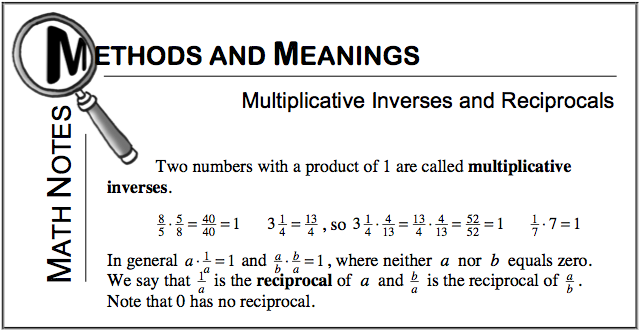### Home > CC2 > Chapter 3 > Lesson 3.2.5 > Problem3-84

3-84.

Read the Math Notes box in this lesson. Use the information to find the multiplicative inverse, or reciprocal, of each number below. Homework Help ✎

1. $\frac { 7 } { 13 }$

See the Math Notes box below.

$\frac{13}{7}$

1. $\frac { 1 } { 5 }$

See part (a).

1. $2$

$2$ can be written as $\frac{2}{1}$

$\frac{1}{2}$

1. $2\frac{5}{8}$

See part (a).

1. Check your answers by multiplying the given number with your answer and verifying that the product of the numbers is $1$.